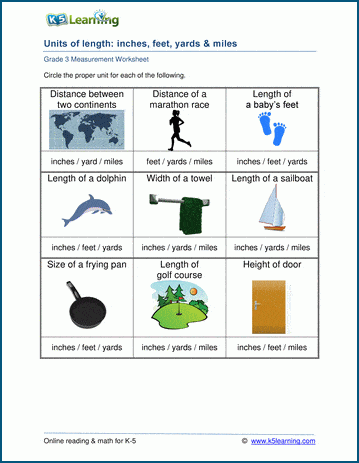# 3rd Grade Math Worksheets: Measurement

Our grade 3 measurement worksheets are designed to help students understand measurements of length, weight, capacity and temperature. Conversion between customary and metric units is also reviewed.

## Lengths

Units of length (inches, feet, yards, miles)

Metric units of length (centimeters, meters and kilometers)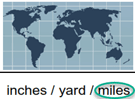Measuring lengths to the nearest quarter inch

Measuring lengths to the nearest millimeter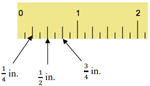Convert between inches, feet, and yards 30 ft = ___ yd
Convert between inches, feet, and yards (harder) 32 ft = ___ yd ___ft

Convert between centimeters and millimeters (no decimals) 80 mm = ____ cm
Convert between centimeters and millimeters  (with decimals) 83 mm = ____ cm
Convert between meters, centimeters and millimeters 8,000 cm = ____ m

## Weights

Reading and using a scale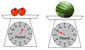Units of weight (ounces and pounds)

Metric units of weight (grams and kilograms)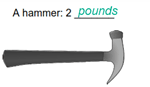Convert ounces and pounds 6 lb = ___ oz
Convert ounces and pounds (harder) 81 oz = ___ lb ___ oz

Convert kilograms & grams 10 kg = ____ g

## Capacities

Reading a measuring cup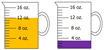Units of capacity (cups, pints, quarts and gallons)

Metric units of capacity (milliliters and liters)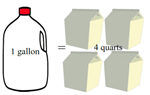Convert cups, pints, quarts, and gallons 28 qt = ___ gal
Convert cups, pints, quarts, and gallons (harder) 14 C = ___ qt ___ C

Convert liters & milliliters 2 l = ____ ml

## Temperatures

Reading a thermometer (Fahrenheit & Celsius)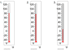Weather and temperature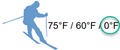## Measurement word problems

Length word problems Word problems
Mass and weight word problems Word problems
Volume and capacity word problems Word problems